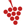{{userInfo.data.fname}} {{userInfo.data.lname}}
{{userInfo.data.company}}
Account Info
Remember me
Not a member? Create an Account
{{userInfo.data.fname}} {{userInfo.data.lname}}
{{userInfo.data.company}}
Account Info
Remember meThe Industry's Leading Publication for Wineries and GrowersThe Industry's Leading Publication for Wineries and Growers

### Calculators

#### Weight and Volume equivalents

Weight equivalents

1 g = 1000 mg
1 mg = 0.001 g
1 µg = 0.001 mg
1 oz = 28.35 g
1 kg = 2.2 lbs = 1000 g
1 lb = 16 oz = 454 g = 0.454 kg
1 (US)ton = 2000 lbs = 907.19 kg
1 metric ton = 2204.62 lbs = 1000 kg

Volume equivalents

1 L = 1000 mL
1 dL = 10 L
1 mL = 1000 µL
1 hL = 100 L = 26.4 gal
1 L = 33.8 oz = 1000 mL
1 (US)gal = 128 oz = 3785 mL = 3.78 L
1 qt = 32 oz = 946 mL = 0.946 L
1 pt = 16 oz = 473 mL = 0.473 L

Weight / Volume equivalents

1.0 g/L = 1000 mg/L = 1000 ppm
1.0 g/L = 0.1 g/100 mL = 100 mg/100 mL
1.0 g/L = 1.00 mg/mL = 1000 µg/mL
1.0 g/L = 0.1%(w/v)
1.0 mg/L = 1ppm
1 lb/1000 gal = 454 g/1000 gal = 0.45 g/gal.
1 lb/1000 gal = 0.12 g/L = 12 g/hL = 120 ppm
1 g/hL = 1 g/26.42 gal. = 0.038 g/gal.Winemaking Calculations powered by VinoEnology.com.